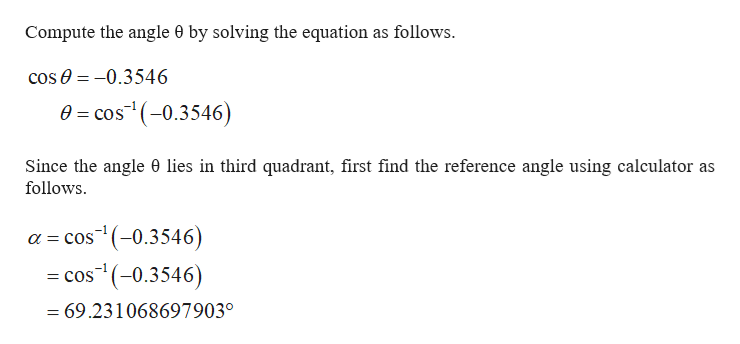# Use the given information and a calculator to find θ to the nearest tenth of a degree, if 0° ≤ θ < 360°.cos θ = −0.3546with θ in QIII

Question
296 views

Use the given information and a calculator to find θ to the nearest tenth of a degree, if 0° ≤ θ < 360°.

cos θ = −0.3546
with θ in QIII
check_circle

Step 1

Consider the trigonometric equation a...help_outlineImage TranscriptioncloseCompute the angle 0 by solving the equation as follows. cose-0.3546 0 cos(-0.3546) Since the angle 0 lies in third quadrant, first find the reference angle using calculator as follows os(-0.3546) a COS os(-0.3546) COS = 69.231068697903° fullscreen

### Want to see the full answer?

See Solution

#### Want to see this answer and more?

Solutions are written by subject experts who are available 24/7. Questions are typically answered within 1 hour.*

See Solution
*Response times may vary by subject and question.
Tagged in

### Trigonometric Ratios